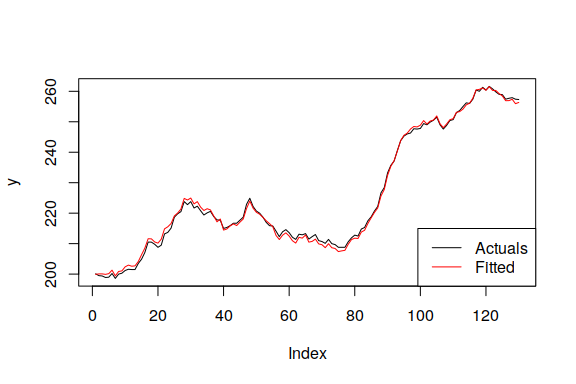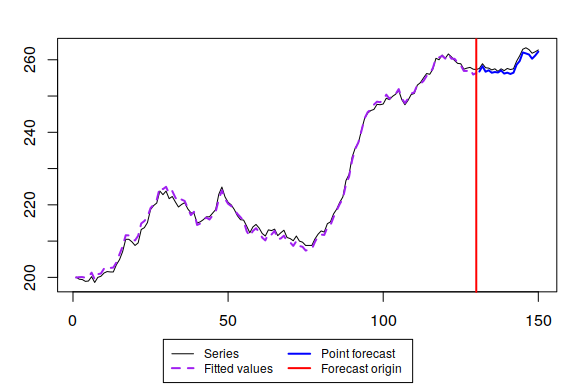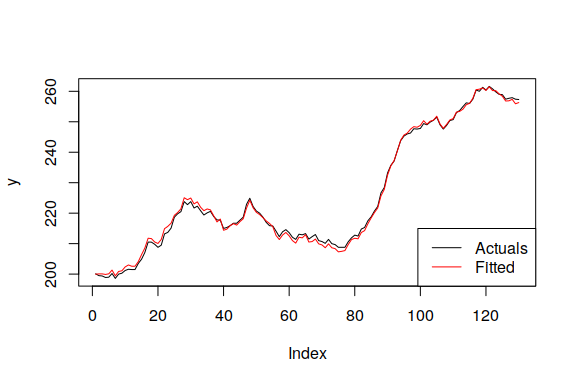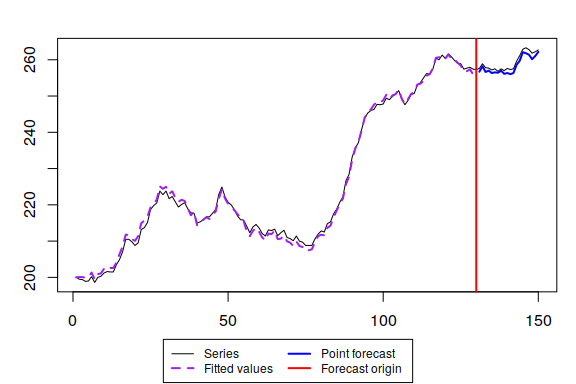# Greybox

#### 2019-04-19

There are three well-known notions of “boxes” in modelling: 1. White box - the model that is completely transparent and does not have any randomness. One can see how the inputs are transformed into the specific outputs. 2. Black box - the model which does not have an apparent structure. One can only observe inputs and outputs but does not know what happens inside. 3. Grey box - the model that is in between the first two. We observe inputs and outputs plus have some information about the structure of the model, but there is still a part of unknown.

The white boxes are usually used in optimisations (e.g. linear programming), while black boxes are popular in machine learning. As for the grey box models, they are more often used in analysis and forecasting. So the package greybox contains models that are used for these purposes.

At the moment the package contains advanced linear model function and several basic functions that implement model selection and combinations using information criteria (IC). You won’t find statistical tests in this package - there’s plenty of them in the other packages. Here we try using the modern techniques and methods that do not rely on hypothesis testing. This is the main philosophical point of greybox.

## Main functions

The package includes the following functions for models construction:

1. alm() - Advanced Linear Model. This is something similar to GLM, but with a focus on forecasting and the information criteria usage for time series. It also supports mixture distribution models for the intermittent data.
2. stepwise() - select the linear model with the lowest IC from all the possible in the provided data. Uses partial correlations. Works fast;
3. lmCombine() - combine the linear models into one using IC weights;
4. lmDynamic() - produce model with dynamic weights and time varying parameters based on IC weight.

See discussion of some of these functions in this vignette below.

## Models evaluation functions

1. ro() - produce forecasts with a specified function using rolling origin.
2. rmc() - regression for multiple comparison of methods. This is a parametric counterpart of nemenyi(), which is more informative than nemenyi() and should work better on large samples. This can be efficiently used together with RelMAE / RelMSE measures.
3. measures() - function, returning a bunch of error measures for the provided forecast and the holdout sample.

## Marketing analytics tools

1. tableplot() - creates a plot for two categorical variables based on table function with frequencies inside.
2. cramer() - Cramer’s V value.
3. mcor() - the multiple correlation coefficient.
4. association() - the matrix of measures of association.
5. spread() - function that plots scatter / boxplot / tableplot diagrams between variables depending on the their types.
6. determination() - coefficients of determination for the set of explanatory variables.

All these functions are discussed in a separate vignette on marketing analytics tools.

## Exogenous variables transformation tools

1. xregExpander() - expand the provided data by including leads and lags of the variables.
2. xregTransformer() - produce non-linear transformations of the provided data (logs, inverse etc).
3. xregMultiplier() - produce cross-products of the variables in the provided matrix. Could be useful when exploring interaction effects of dummy variables.

See details on (1) below.

## Distribution functions

1. qlaplace(), dlaplace(), rlaplace(), plaplace() - functions for Laplace distribution.
2. qalaplace(), dalaplace(), ralaplace(), palaplace() - functions for Asymmetric Laplace distribution.
3. qs(), ds(), rs(), ps() - functions for S distribution.
4. qfonrm(), dfnorm(), rfnorm(), pfnorm() - functions for folded normal distribution.
5. qtplnorm(), dtplnorm(), rtplnorm(), ptplnorm() - functions for three parameter log normal distribution.

1. graphmaker() - produces linear plots for the variable, its forecasts and fitted values.

The first two construct a model of a class lm, that could be used for the purposes of analysis or forecasting. The last one expands the exogenous variables to the matrix with lags and leads. Let’s see how all of them work. Let’s start from the end.

## xregExpander

The function xregExpander() is useful in cases when the exogenous variable may influence the response variable either via some lags or leads. As an example, consider BJsales.lead series from the datasets package. Let’s assume that the BJsales variable is driven by the today’s value of the indicator, the value five and 10 days ago. This means that we need to produce lags of BJsales.lead. This can be done using xregExpander():

BJxreg <- xregExpander(BJsales.lead,lags=c(-5,-10))

The BJxreg is a matrix, which contains the original data, the data with the lag 5 and the data with the lag 10. However, if we just move the original data several observations ahead or backwards, we will have missing values in the beginning / end of series, so xregExpander() fills in those values with the forecasts using es() and iss() functions from smooth package (depending on the type of variable we are dealing with). This also means that in cases of binary variables you may have weird averaged values as forecasts (e.g. 0.7812), so beware and look at the produced matrix. Maybe in your case it makes sense to just substitute these weird numbers with zeroes…

You may also need leads instead of lags. This is regulated with the same lags parameter but with positive values:

BJxreg <- xregExpander(BJsales.lead,lags=c(7,-5,-10))

Once again, the values are shifted, and now the first 7 values are backcasted. In order to simplify things we can produce all the values from 10 lags till 10 leads, which returns the matrix with 21 variables:

BJxreg <- xregExpander(BJsales.lead,lags=c(-10:10))

## stepwise

The function stepwise() does the selection based on an information criterion (specified by user) and partial correlations. In order to run this function the response variable needs to be in the first column of the provided matrix. The idea of the function is simple, it works iteratively the following way:

1. The basic model of the first variable and the constant is constructed (this corresponds to simple mean). An information criterion is calculated;
2. The correlations of the residuals of the model with all the original exogenous variables are calculated;
3. The regression model of the response variable and all the variables in the previous model plus the new most correlated variable from (2) is constructed using lm() function;
4. An information criterion is calculated and is compared with the one from the previous model. If it is greater or equal to the previous one, then we stop and use the previous model. Otherwise we go to step 2.

This way we do not do a blind search, going forward or backwards, but we follow some sort of “trace” of a good model: if the residuals contain a significant part of variance that can be explained by one of the exogenous variables, then that variable is included in the model. Following partial correlations makes sure that we include only meaningful (from technical point of view) variables in the model. In general the function guarantees that you will have the model with the lowest information criterion. However this does not guarantee that you will end up with a meaningful model or with a model that produces the most accurate forecasts. So analyse what you get as a result.

Let’s see how the function works with the Box-Jenkins data. First we expand the data and form the matrix with all the variables:

BJxreg <- as.data.frame(xregExpander(BJsales.lead,lags=c(-10:10)))
BJxreg <- cbind(as.matrix(BJsales),BJxreg)
colnames(BJxreg) <- "y"
ourModel <- stepwise(BJxreg)

This way we have a nice data frame with nice names, not something weird with strange long names. It is important to note that the response variable should be in the first column of the resulting matrix. After that we use stepwise function:

ourModel <- stepwise(BJxreg)

And here’s what it returns (the object of class lm):

ourModel
#> Call:
#> alm(formula = y ~ xLag4 + xLag9 + xLag3 + xLag10 + xLag5 + xLag6 +
#>     xLead9 + xLag7 + xLag8, data = data, distribution = "dnorm")
#>
#> Coefficients:
#> (Intercept)       xLag4       xLag9       xLag3      xLag10       xLag5
#>  17.6448130   3.3712172   1.3724166   4.6781047   1.5412073   2.3213096
#>   1.7075130   0.3766691   1.4024773   1.3370199

The values in the function are listed in the order of most correlated with the response variable to the least correlated ones. The function works very fast because it does not need to go through all the variables and their combinations in the dataset.

All the basic methods can be used together with the final model (e.g. predict(), forecast(), summary() etc).

## lmCombine

lmCombine() function creates a pool of linear models using lm(), writes down the parameters, standard errors and information criteria and then combines the models using IC weights. The resulting model is of the class “lm.combined”. The speed of the function deteriorates exponentially with the increase of the number of variables $$k$$ in the dataset, because the number of combined models is equal to $$2^k$$. The advanced mechanism that uses stepwise() and removes a large chunk of redundant models is also implemented in the function and can be switched using bruteForce parameter.

Here’s an example of the reduced data with combined model and the parameter bruteForce=TRUE:

ourModel <- lmCombine(BJxreg[,-c(3:7,18:22)],bruteForce=TRUE)
summary(ourModel)
#> Response variable: y
#> Distribution used in the estimation: Normal
#> Coefficients:
#>             Estimate Std. Error Importance Lower 2.5% Upper 97.5%
#> (Intercept) 20.90312    1.81649      1.000   17.31243    24.49380
#> x           -0.04283    0.20934      0.256   -0.45663     0.37097
#> xLag5        6.39707    0.65301      1.000    5.10626     7.68788
#> xLag4        5.84667    0.70751      1.000    4.44812     7.24522
#> xLag3        5.68545    0.72261      1.000    4.25704     7.11385
#> xLag2        0.12328    0.31266      0.284   -0.49476     0.74132
#> xLag1       -0.08344    0.26044      0.269   -0.59825     0.43138
#> xLead1      -0.08953    0.25863      0.275   -0.60077     0.42170
#> xLead2      -0.03508    0.19264      0.257   -0.41587     0.34570
#> xLead3      -0.11763    0.28383      0.293   -0.67868     0.44341
#> xLead4      -0.00672    0.15873      0.256   -0.32048     0.30704
#> xLead5       0.11405    0.26333      0.300   -0.40647     0.63457
#> ---
#> Residual standard error: 2.20758 on 142.81 degrees of freedom:
#> Approximate combined ICs:
#>      AIC     AICc      BIC     BICc
#> 670.6390 671.4695 692.2854 694.3661
#>
#> Sample size: 150
#> Number of estimated parameters: 7.19
#> Number of degrees of freedom: 142.81

summary() function provides the table with the parameters, their standard errors, their relative importance and the 95% confidence intervals. Relative importance indicates in how many cases the variable was included in the model with high weight. So, in the example above variables xLag5, xLag4, xLag3 were included in the models with the highest weights, while all the others were in the models with lower ones. This may indicate that only these variables are needed for the purposes of analysis and forecasting.

The more realistic situation is when the number of variables is high. In the following example we use the data with 21 variables. So if we use brute force and estimate every model in the dataset, we will end up with $$2^{21}$$ = 2^21 combinations of models, which is not possible to estimate in the adequate time. That is why we use bruteForce=FALSE:

ourModel <- lmCombine(BJxreg,bruteForce=FALSE)
summary(ourModel)
#> Response variable: y
#> Distribution used in the estimation: Normal
#> Coefficients:
#>             Estimate Std. Error Importance Lower 2.5% Upper 97.5%
#> (Intercept) 17.67087    0.81312      1.000   16.06320    19.27854
#> xLag4        3.37561    0.31398      1.000    2.75481     3.99641
#> xLag9        1.37089    0.31459      1.000    0.74889     1.99289
#> xLag3        4.68607    0.29355      1.000    4.10568     5.26646
#> xLag10       1.54203    0.28533      1.000    0.97790     2.10617
#> xLag5        2.32253    0.32349      1.000    1.68293     2.96212
#> xLag6        1.70763    0.32629      1.000    1.06250     2.35276
#> xLead9       0.36369    0.13677      0.966    0.09327     0.63411
#> xLag7        1.40141    0.32736      1.000    0.75416     2.04866
#> xLag8        1.33615    0.32561      0.999    0.69237     1.97993
#> ---
#> Residual standard error: 0.93694 on 139.035 degrees of freedom:
#> Approximate combined ICs:
#>      AIC     AICc      BIC     BICc
#> 417.0047 418.9056 450.0163 454.7787
#>
#> Sample size: 150
#> Number of estimated parameters: 10.965
#> Number of degrees of freedom: 139.035

In this case first, the stepwise() function is used, which finds the best model in the pool. Then each variable that is not in the model is added to the model and then removed iteratively. IC, parameters values and standard errors are all written down for each of these expanded models. Finally, in a similar manner each variable is removed from the optimal model and then added back. As a result the pool of combined models becomes much smaller than it could be in case of the brute force, but it contains only meaningful models, that are close to the optimal. The rationale for this is that the marginal contribution of variables deteriorates with the increase of the number of parameters in case of the stepwise function, and the IC weights become close to each other around the optimal model. So, whenever the models are combined, there is a lot of redundant models with very low weights. By using the mechanism described above we remove those redundant models.

There are several methods for the lm.combined class, including:

1. predict.greybox() - returns the point and interval predictions.
2. forecast.greybox() - wrapper around predict() The forecast horizon is defined by the length of the provided sample of newdata.
3. plot.lm.combined() - plots actuals and fitted values.
4. plot.predict.greybox() - which uses graphmaker() function from smooth in order to produce graphs of actuals and forecasts.

As an example, let’s split the whole sample with Box-Jenkins data into in-sample and the holdout:

BJInsample <- BJxreg[1:130,];
BJHoldout <- BJxreg[-(1:130),];
ourModel <- lmCombine(BJInsample,bruteForce=FALSE)

A summary and a plot of the model:

summary(ourModel)
#> Response variable: y
#> Distribution used in the estimation: Normal
#> Coefficients:
#>             Estimate Std. Error Importance Lower 2.5% Upper 97.5%
#> (Intercept) 19.39048    0.97639      1.000   17.45714    21.32382
#> xLag4        3.34935    0.33360      1.000    2.68880     4.00990
#> xLag9        1.33366    0.33590      0.999    0.66855     1.99878
#> xLag3        4.75814    0.31641      1.000    4.13162     5.38467
#> xLag10       1.53628    0.30330      1.000    0.93571     2.13685
#> xLag5        2.32134    0.34360      1.000    1.64100     3.00169
#> xLag6        1.66121    0.34661      1.000    0.97489     2.34752
#> xLead9       0.29376    0.15498      0.889   -0.01311     0.60063
#> xLag8        1.36901    0.34715      0.999    0.68162     2.05640
#> xLag7        1.32692    0.34869      0.998    0.63649     2.01735
#> ---
#> Residual standard error: 0.9554 on 119.115 degrees of freedom:
#> Approximate combined ICs:
#>      AIC     AICc      BIC     BICc
#> 368.1714 370.3620 399.3845 404.7158
#>
#> Sample size: 130
#> Number of estimated parameters: 10.885
#> Number of degrees of freedom: 119.115
plot(ourModel)Importance tells us how important the respective variable is in the combination. 1 means 100% important, 0 means not important at all.

And the forecast using the holdout sample:

ourForecast <- predict(ourModel,BJHoldout)
#> Warning: The covariance matrix for combined models is approximate. Don't
#> rely too much on that.
plot(ourForecast)These are the main functions implemented in the package for now. If you want to read more about IC model selection and combinations, I would recommend (Burnham and Anderson 2004) textbook.

## lmDynamic

This function is based on the principles of lmCombine() and point ICs. It allows not only combining the models but also to capture the dynamics of it parameters. So in a way this corresponds to a time varying parameters model, but based on information criteria.

Continuing the example from lmCombine(), let’s construct the dynamic model:

ourModel <- lmDynamic(BJInsample,bruteForce=FALSE)

We can plot the model and ask for the summary in the similar way as with lmCombine():

ourSummary <- summary(ourModel)
ourSummary
#> Response variable: y
#> Distribution used in the estimation: Normal
#> Coefficients:
#>             Estimate Std. Error Importance Lower 2.5% Upper 97.5%
#> (Intercept) 19.74382    1.12400    1.00000   17.51847    21.96917
#> xLag4        3.50174    0.52297    0.97718    2.46635     4.53713
#> xLag9        1.34289    0.72632    0.79844   -0.09511     2.78089
#> xLag3        4.88153    0.48796    0.98466    3.91545     5.84761
#> xLag10       1.55220    0.67730    0.83981    0.21126     2.89314
#> xLag5        2.30847    0.66468    0.90440    0.99251     3.62443
#> xLag6        1.62346    0.70395    0.83863    0.22976     3.01717
#> xLead9       0.20167    0.20522    0.59208   -0.20464     0.60798
#> xLag8        1.28494    0.74929    0.76283   -0.19852     2.76841
#> xLag7        1.22305    0.73469    0.75564   -0.23152     2.67761
#> ---
#> Residual standard error: 0.95505 on 120.54633 degrees of freedom:
#> Approximate combined ICs:
#>      AIC     AICc      BIC     BICc
#> 394.9237 396.5770 422.0324 426.0562
#>
#> Sample size: 130
#> Number of estimated parameters: 9.453667
#> Number of degrees of freedom: 120.5463
plot(ourModel)The coefficients in the summary are the averaged out over the whole sample. The more interesting elements are the time varying parameters, their standard errors (and respective confidence intervals) and time varying importance of the parameters.

# Coefficients in dynamics
head(ourModel$coefficientsDynamic) #> (Intercept) xLag4 xLag9 xLag3 xLag10 xLag5 xLag6 #> [1,] 19.78555 3.405125 1.305010 4.86535320 1.5453547 2.336761 1.657519 #> [2,] 19.52654 3.375886 1.316125 4.80214807 1.5713704 2.345914 1.676755 #> [3,] 19.82085 3.429083 1.275137 4.89384101 1.6318047 2.390156 1.695951 #> [4,] 20.12319 3.632261 1.094000 5.16064553 2.0729802 2.725020 1.367984 #> [5,] 20.10123 3.798097 1.548715 5.11105846 2.1349674 2.740274 1.247352 #> [6,] 19.25164 5.248581 1.662278 0.02359287 0.9432015 3.673436 2.524485 #> xLead9 xLag8 xLag7 #> [1,] 1.276469e-01 1.3664495 1.3100757 #> [2,] 2.378845e-01 1.3473942 1.2655912 #> [3,] 1.166030e-01 1.3167440 1.1668648 #> [4,] 2.566841e-05 1.4007476 0.4378367 #> [5,] 1.194837e-08 0.5170024 0.7960640 #> [6,] 8.739561e-01 0.7380312 2.2717602 # Standard errors of the coefficients in dynamics head(ourModel$se)
#>      (Intercept)     xLag4     xLag9     xLag3    xLag10     xLag5
#> [1,]    1.005564 0.3516119 0.3440133 0.3240634 0.3076014 0.3488008
#> [2,]    1.033214 0.3632487 0.3727267 0.3370008 0.3196004 0.3530545
#> [3,]    1.018598 0.3576335 0.4361379 0.3335079 0.3491827 0.3709207
#> [4,]    1.091927 0.4346210 0.8218602 0.4502489 0.6584449 0.6163140
#> [5,]    1.105609 0.5039341 0.7694555 0.4317954 0.7431743 0.6324804
#> [6,]    1.719871 1.8259868 0.7767458 4.8589065 0.8467085 1.4801397
#> [1,] 0.3523658 0.1951738 0.3624849 0.3668672
#> [2,] 0.3616669 0.1891749 0.4029963 0.4214938
#> [3,] 0.3868707 0.1951469 0.4760700 0.5126188
#> [4,] 0.8697687 0.2016674 0.8741159 1.0697642
#> [5,] 1.0164058 0.2016700 1.1347675 1.0140215
#> [6,] 1.0775806 0.7092682 0.8846370 1.2089227
# Importance of parameters in dynamics
head(ourModel$importance) #> (Intercept) xLag4 xLag9 xLag3 xLag10 xLag5 #> [1,] 1 1.0000000 0.9967486 1.000000000 0.9999732 1.0000000 #> [2,] 1 1.0000000 0.9731689 1.000000000 0.9995738 0.9999992 #> [3,] 1 1.0000000 0.9257820 1.000000000 0.9985305 0.9999931 #> [4,] 1 0.9999998 0.6446641 1.000000000 0.9926558 0.9900452 #> [5,] 1 1.0000000 0.8326000 1.000000000 0.9783812 0.9904669 #> [6,] 1 1.0000000 0.9317261 0.005152122 0.7561710 1.0000000 #> xLag6 xLead9 xLag8 xLag7 #> [1,] 0.9998997 3.864048e-01 0.9975854 0.9955111 #> [2,] 0.9976606 7.236236e-01 0.9656598 0.9427636 #> [3,] 0.9909696 3.582759e-01 0.9140867 0.8542494 #> [4,] 0.6910754 9.600656e-05 0.7391000 0.2567121 #> [5,] 0.5987277 5.124769e-08 0.2723966 0.4318514 #> [6,] 0.9993044 9.942779e-01 0.5951329 0.9961069 The importance can also be plotted using plot() and coef() functions, which might produce a lot of images: The plots show how the importance of each parameter changes over time. The values do not look as smooth as we would like them to, but nothing can be done with this at this point. If you want something smooth, then smooth these values out using, for example, cma() function from smooth package. In fact, even degrees of freedom are now also time varying: ourModel$dfDynamic
#>    10.376123 10.602450 10.041887  8.314348  8.104424  9.277871  8.580929
#>     9.902191  9.366828  8.074966  7.459910  8.043190  8.032306  9.072062
#>    7.065215  7.001421  8.923675  7.841104  9.182772  8.944887  7.679829
#>    8.992845  9.771926  9.980439 10.590096 10.947603  9.999912  9.856056
#>    8.999852  9.930299  9.661263  8.989051  9.349294  8.665523  9.005119
#>    9.621022 10.983185  9.282105 10.050140  9.396438  9.320468 10.736370
#>   10.064395  9.998790  9.991561  9.996159  8.983045  8.998081  9.949832
#>    9.963220  9.956193 10.850813  9.650169  9.961525 10.100268  9.327175
#>    8.676455  8.971486  8.989004  8.563124  8.091551  8.155178  8.322737
#>    8.731393  9.902151  8.390217  9.676468  8.451362  8.482846  8.607932
#>    8.708372  8.907144  8.990101  8.754263  8.060967  9.355366  8.911087
#>    9.040374  9.980193  8.996481  9.001699  9.000132  9.893101  9.974498
#>   10.580089 10.000910  9.998900  8.950899  9.984999  8.713775 10.153345
#>   10.633372 10.966921 10.985145 10.940877 10.464586  8.082171  9.748390
#>    9.032544  8.223434  8.967503 10.046643  9.904137 10.871078  9.965905
#>   9.988085  9.985907  9.347830 10.009423 10.099447 10.783947  9.816844
#>   9.160745  9.803834 10.776737 10.348301 10.983367  9.975085 10.404917
#>  10.933127 10.787225  9.781041  9.633336 10.501708  9.724648  9.947751
#>   8.617128  9.625946  8.525336  8.805082
ourModel\$df.residualDynamic
#>    119.6239 119.3976 119.9581 121.6857 121.8956 120.7221 121.4191
#>    120.0978 120.6332 121.9250 122.5401 121.9568 121.9677 120.9279
#>   122.9348 122.9986 121.0763 122.1589 120.8172 121.0551 122.3202
#>   121.0072 120.2281 120.0196 119.4099 119.0524 120.0001 120.1439
#>   121.0001 120.0697 120.3387 121.0109 120.6507 121.3345 120.9949
#>   120.3790 119.0168 120.7179 119.9499 120.6036 120.6795 119.2636
#>   119.9356 120.0012 120.0084 120.0038 121.0170 121.0019 120.0502
#>   120.0368 120.0438 119.1492 120.3498 120.0385 119.8997 120.6728
#>   121.3235 121.0285 121.0110 121.4369 121.9084 121.8448 121.6773
#>   121.2686 120.0978 121.6098 120.3235 121.5486 121.5172 121.3921
#>   121.2916 121.0929 121.0099 121.2457 121.9390 120.6446 121.0889
#>   120.9596 120.0198 121.0035 120.9983 120.9999 120.1069 120.0255
#>   119.4199 119.9991 120.0011 121.0491 120.0150 121.2862 119.8467
#>   119.3666 119.0331 119.0149 119.0591 119.5354 121.9178 120.2516
#>   120.9675 121.7766 121.0325 119.9534 120.0959 119.1289 120.0341
#>  120.0119 120.0141 120.6522 119.9906 119.9006 119.2161 120.1832
#>  120.8393 120.1962 119.2233 119.6517 119.0166 120.0249 119.5951
#>  119.0669 119.2128 120.2190 120.3667 119.4983 120.2754 120.0522
#>  121.3829 120.3741 121.4747 121.1949

And as usual we can produce forecast from this function, the mean parameters are used in this case:

ourForecast <- predict(ourModel,BJHoldout)
#> Warning: The covariance matrix for combined models is approximate. Don't
#> rely too much on that.
plot(ourForecast)This function is currently under development, so stay tuned.